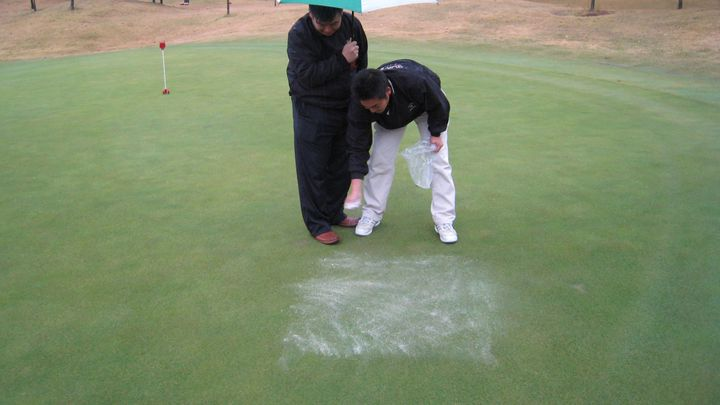# Calcium for turfgrass: is there enough in the soil?Putting out a test of calcium magnesium carbonate.

The answer, almost certainly, is yes. These calculations of how much calcium the grass uses, compared to how much plant-available calcium is in the soil, leave little doubt that there is almost always more than enough calcium in the soil to meet the needs of the grass.

## How much calcium does grass use?

Let’s take a turfgrass site at which clippings are removed, and estimate how much calcium is removed, or harvested, in one year. A reasonable estimate for annual clipping yield of maintained turfgrass in a temperate climate is about 400 g m-2. A reasonable estimate for calcium in the dry matter of turfgrass clippings is about 4500 ppm = 4.5 g kg-1 = 0.45%. So if we harvest, over the course of a year, 400 grams of clippings from each square meter, and if those clippings contain on average 0.45% calcium, then the mass of calcium harvested from one square meter each year is:

400 g x 0.0045 = 1.8 grams of calcium removed each year

## How much calcium is in the soil?

We’ve just calculated that in the situation where we remove the clippings from a maintained turfgrass area, we may be removing about 1.8 grams of calcium from each square meter each year. Let’s now imagine that our root system is 10 cm deep, and that the bulk density of the soil is 1.5 g cm-3. In one square meter, then, to a depth of 10 cm, we have a volume of 100 L, and a mass of 150 kg. Let’s say this is a sandy soil, with a relatively low cation exchange capacity, and that the plant-available calcium (the calcium that is in soil solution and on cation exchange sites) is at a concentration of 400 ppm = 400 mg kg-1. How much calcium is that?

150 kg of soil x 400 mg/kg = 60,000 mg of calcium = 60 g of calcium